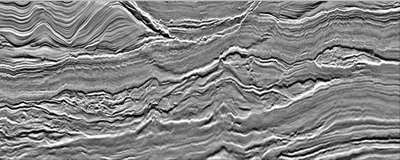# Imaging the earth with sound waves: basic principles of reflection

Added: 8th August 2019 by CGG

When you look at a seismic image of the earth generated from reflection data, what is actually imaged are the interfaces or boundaries between layers rather than the rock layers themselves.When exposed at the surface a rock may be very strongly layered, but if it is buried what governs whether we observe that layering is the signal bandwidth, which controls the resolution of our image; and the contrast in seismic impedance Z between the different layers, which determines whether a reflection occurs and its amplitude. Seismic impedance Z is the product of the seismic wave velocity v and the density of the medium ?.

Z=v*p

For the simplest case of a seismic wave vertically incident at the boundary between two flat layers the amplitude of the reflected wave can be calculated using the reflection co-efficient R multiplied by the amplitude of the incident wave.

R=(Z2-Z1)/(Z2+Z1)

R is Z2 minus Z1 divided by the sum of Z1 and Z2; where Z1 is the seismic impedance of the layer or medium the wave is leaving and Z2 is the seismic impedance of the layer the wave is entering. So for the common scenario of a seismic wave vertically incident at the sea floor, assuming typical values of density and velocity of 1000 kg/m³ and 1500 m/s for water, and a rocky seabed with density 2000 kg/m³ and velocity of 2000 m/s, the reflection co-efficient is ~0.45. Using this information we can make inferences about rock types, fluid content and whether a recorded signal is geological in origin or an effect of our data acquisition.